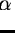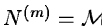Next: Test statistics Up: Basic principles of time Previous: Signals and their models

Signal detection

Paradoxically, signal detection is concerned with fitting models to supposedly random series similarly to mathematical proofs by reductio ad absurdum of the antithesis. That is, the hypothesis (antithesis) Ho is made, that the observed series X(o) has properties of a pure noise series, N(o). Then, a model is fitted and series X(m) and X(r) are obtained. If the quality of the model fits to the observations X(o) does not significantly differ from the quality of a fit to pure noise N(o), then Ho is true and we say that X(o) contains no signal but noise. In the opposite case of model fitting X(o) significantly better than N(o), we reject Ho and say that the model signal was detected in X(o). The difference is significant (at some level) if it is not likely (at this level) to occur between two different realizations of the noise N(o).

The quality of the fit is evaluated using a function S of the series X(o), X(m), and X(r). A function of random variables, such as S(X(o)), is a random variable itself and is called a statistic. A random variable S is characterized by its probability distribution function. Following Ho we use the distribution of Sfor pure noise signal N(o), N(m) and N(r), to be denoted pN(S) or simply p(S). Precisely, we shall use the cumulative probability distribution function which for a given critical value of the statistic S=So supplies the probability p(So) for the observed S to fall on one side of the So.

The observed value of the statistic and its probability distribution, S(X(o)) and p(S) respectively, are used to obtain the probability p(S(X)) of Ho being true. That is, if p turns out small,, Ho is improbable and X(o) has no properties of N(o). Then we say that the model signal has been detected at the confidence level. The smalleris, the more convincing (significant) is the detection. The special realization of a random series which consists of independent variables of common (gaussian) distribution is called (gaussian) white noise. We assume here that the noise N(o) is white noise. Note that in the signal detection process, frequencyand lag l are considered independent variables and do not count as parameters.

Summarizing, the basis for the determination of the properties of a an observed time series is a test statistic, S, with known probability distribution for (white) noise, p(S).

Let N(o) consist of no random variables and let a given model have nm parameters. Then the modeled series N(m) corresponds to a combination of nm random variables and the residual series N(r) corresponds to a combination of nr=no-nm random variables. The proof rests on the observation that orthogonal transformations convert vectors of independent variables into vectors of independent variables. Let us consider an approximately linear model with matrixso that, where P is a vector of nm parameters. Then N(m) spans a vector space with no more than nm orthogonal vectors (dimensions). The numbers no, nm and nr are called the numbers of degrees of freedom of the observations, the model fit, and the residuals, respectively.Next: Test statistics Up: Basic principles of time Previous: Signals and their models
Petra Nass
1999-06-15